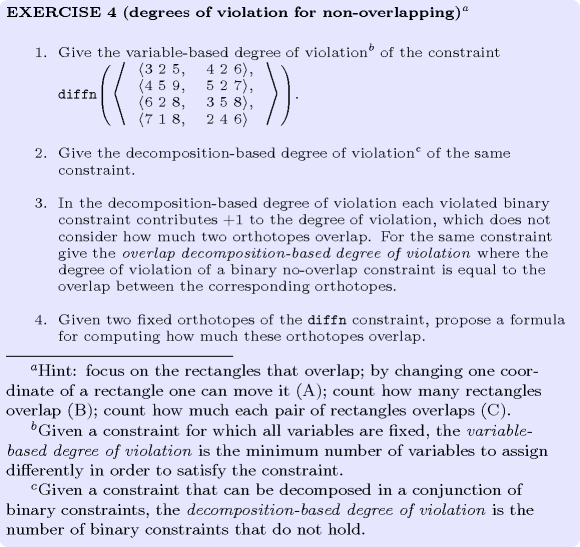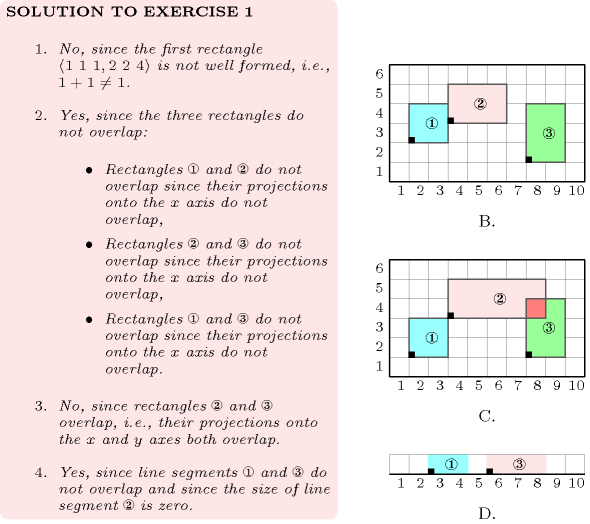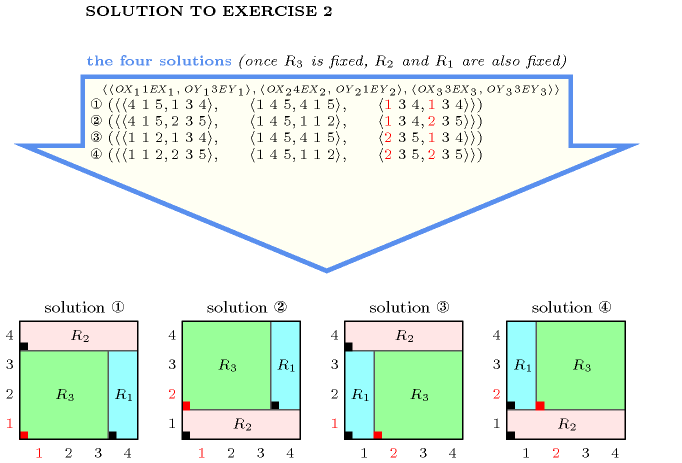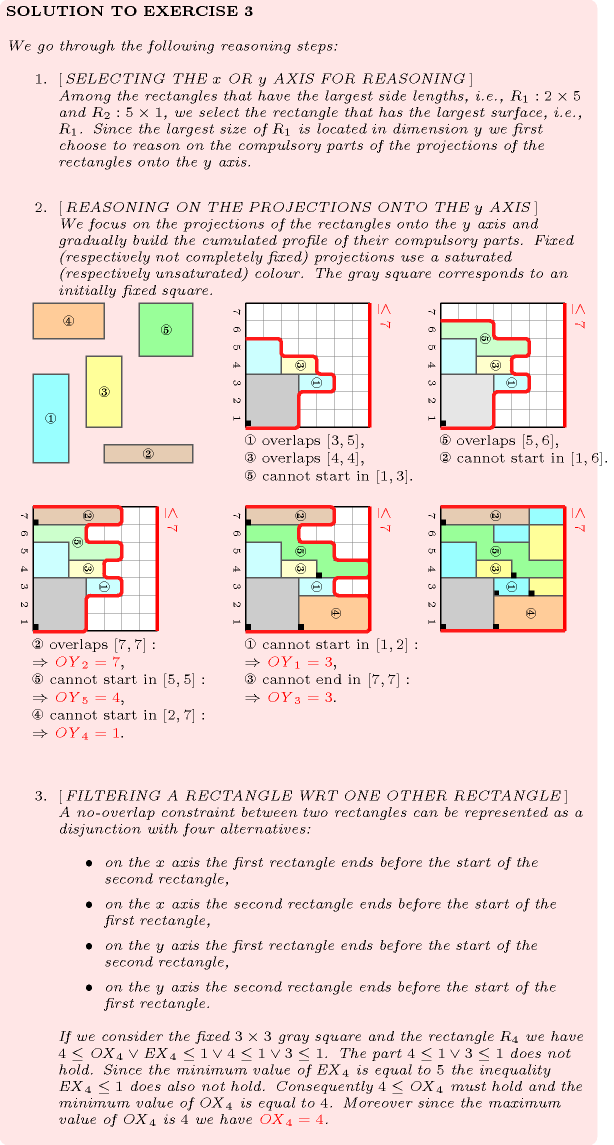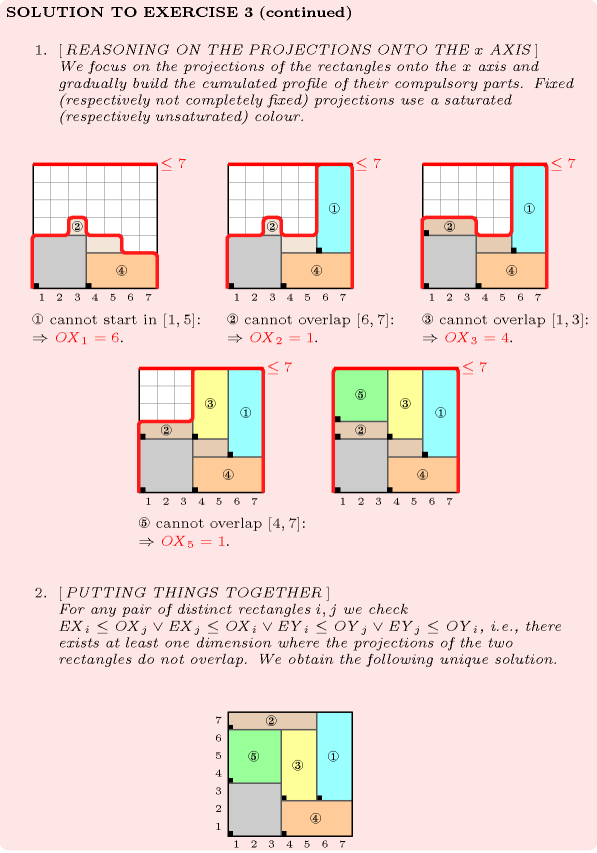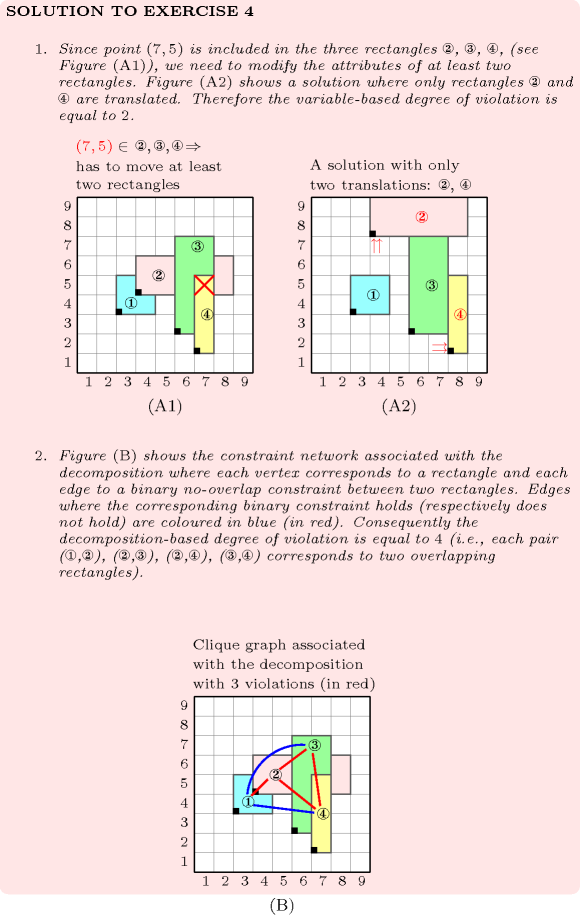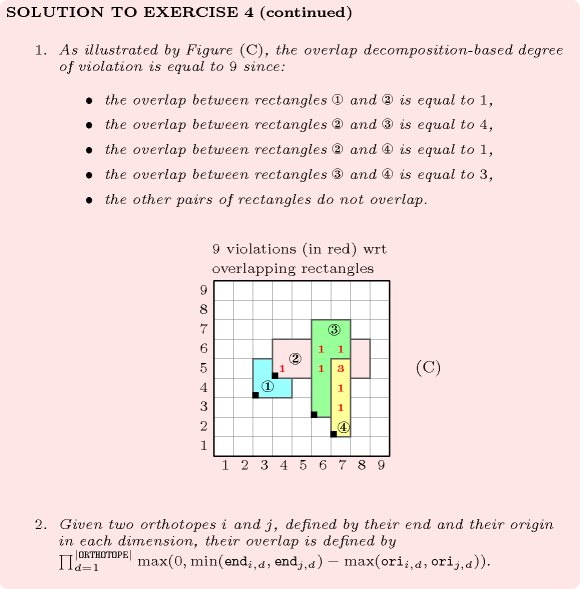## 5.118. diffn

Origin
Constraint

$\mathrm{𝚍𝚒𝚏𝚏𝚗}\left(\mathrm{𝙾𝚁𝚃𝙷𝙾𝚃𝙾𝙿𝙴𝚂}\right)$

Synonyms

$\mathrm{𝚍𝚒𝚜𝚓𝚘𝚒𝚗𝚝}$, $\mathrm{𝚍𝚒𝚜𝚓𝚘𝚒𝚗𝚝}\mathtt{1}$, $\mathrm{𝚍𝚒𝚜𝚓𝚘𝚒𝚗𝚝}\mathtt{2}$, $\mathrm{𝚍𝚒𝚏𝚏}\mathtt{2}$.

Type
 $\mathrm{𝙾𝚁𝚃𝙷𝙾𝚃𝙾𝙿𝙴}$ $\mathrm{𝚌𝚘𝚕𝚕𝚎𝚌𝚝𝚒𝚘𝚗}\left(\mathrm{𝚘𝚛𝚒}-\mathrm{𝚍𝚟𝚊𝚛},\mathrm{𝚜𝚒𝚣}-\mathrm{𝚍𝚟𝚊𝚛},\mathrm{𝚎𝚗𝚍}-\mathrm{𝚍𝚟𝚊𝚛}\right)$
Argument
 $\mathrm{𝙾𝚁𝚃𝙷𝙾𝚃𝙾𝙿𝙴𝚂}$ $\mathrm{𝚌𝚘𝚕𝚕𝚎𝚌𝚝𝚒𝚘𝚗}\left(\mathrm{𝚘𝚛𝚝𝚑}-\mathrm{𝙾𝚁𝚃𝙷𝙾𝚃𝙾𝙿𝙴}\right)$
Restrictions
 $|\mathrm{𝙾𝚁𝚃𝙷𝙾𝚃𝙾𝙿𝙴}|>0$ $\mathrm{𝚛𝚎𝚚𝚞𝚒𝚛𝚎}_\mathrm{𝚊𝚝}_\mathrm{𝚕𝚎𝚊𝚜𝚝}$$\left(2,\mathrm{𝙾𝚁𝚃𝙷𝙾𝚃𝙾𝙿𝙴},\left[\mathrm{𝚘𝚛𝚒},\mathrm{𝚜𝚒𝚣},\mathrm{𝚎𝚗𝚍}\right]\right)$ $\mathrm{𝙾𝚁𝚃𝙷𝙾𝚃𝙾𝙿𝙴}.\mathrm{𝚜𝚒𝚣}\ge 0$ $\mathrm{𝙾𝚁𝚃𝙷𝙾𝚃𝙾𝙿𝙴}.\mathrm{𝚘𝚛𝚒}\le \mathrm{𝙾𝚁𝚃𝙷𝙾𝚃𝙾𝙿𝙴}.\mathrm{𝚎𝚗𝚍}$ $\mathrm{𝚛𝚎𝚚𝚞𝚒𝚛𝚎𝚍}$$\left(\mathrm{𝙾𝚁𝚃𝙷𝙾𝚃𝙾𝙿𝙴𝚂},\mathrm{𝚘𝚛𝚝𝚑}\right)$ $\mathrm{𝚜𝚊𝚖𝚎}_\mathrm{𝚜𝚒𝚣𝚎}$$\left(\mathrm{𝙾𝚁𝚃𝙷𝙾𝚃𝙾𝙿𝙴𝚂},\mathrm{𝚘𝚛𝚝𝚑}\right)$
Purpose

Generalised multi-dimensional non-overlapping constraint: Holds if, for each pair of orthotopes $\left({O}_{1},{O}_{2}\right)$, ${O}_{1}$ and ${O}_{2}$ do not overlap. Two orthotopes do not overlap if one of the orthotopes has zero size or if there exists at least one dimension where their projections do not overlap.

Example
$\left(\begin{array}{c}〈\begin{array}{c}\mathrm{𝚘𝚛𝚝𝚑}-〈\mathrm{𝚘𝚛𝚒}-2\mathrm{𝚜𝚒𝚣}-2\mathrm{𝚎𝚗𝚍}-4,\mathrm{𝚘𝚛𝚒}-1\mathrm{𝚜𝚒𝚣}-2\mathrm{𝚎𝚗𝚍}-3〉,\hfill \\ \mathrm{𝚘𝚛𝚝𝚑}-〈\mathrm{𝚘𝚛𝚒}-4\mathrm{𝚜𝚒𝚣}-4\mathrm{𝚎𝚗𝚍}-8,\mathrm{𝚘𝚛𝚒}-2\mathrm{𝚜𝚒𝚣}-2\mathrm{𝚎𝚗𝚍}-4〉,\hfill \\ \mathrm{𝚘𝚛𝚝𝚑}-〈\begin{array}{ccc}\mathrm{𝚘𝚛𝚒}-6\hfill & \mathrm{𝚜𝚒𝚣}-5\hfill & \mathrm{𝚎𝚗𝚍}-11,\hfill \\ \mathrm{𝚘𝚛𝚒}-5\hfill & \mathrm{𝚜𝚒𝚣}-2\hfill & \mathrm{𝚎𝚗𝚍}-7\hfill \end{array}〉\hfill \end{array}〉\hfill \end{array}\right)$

Figure 5.118.1 represents the position of the three rectangles of the example. The coordinates of the leftmost lowest corner of each rectangle are stressed in bold. The $\mathrm{𝚍𝚒𝚏𝚏𝚗}$ constraint holds since the three rectangles do not overlap as explained in Figure 5.118.2.

##### Figure 5.118.1. Illustration of the Example slot: the three rectangles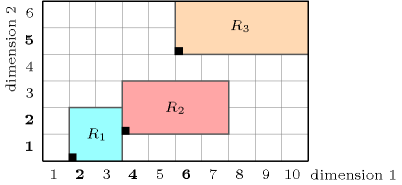##### Figure 5.118.2. Illustration of the Example slot: the reasons (A), (B), (C) why the pairs of rectangles $\left({R}_{1},{R}_{2}\right)$, $\left({R}_{1},{R}_{3}\right)$, $\left({R}_{2},{R}_{3}\right)$ do not overlap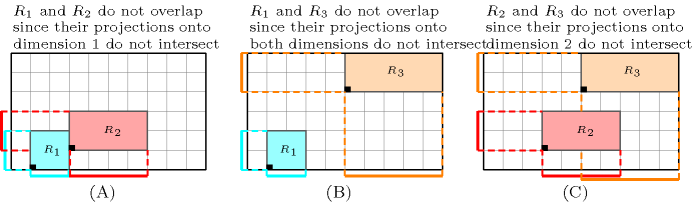All solutions

Figure 5.118.3 gives all solutions to the following non ground instance of the $\mathrm{𝚍𝚒𝚏𝚏𝚗}$ constraint:

${X}_{1}\in \left[1,3\right]$, ${\mathrm{𝐸𝑋}}_{1}\in \left[1,9\right]$, ${Y}_{1}\in \left[1,3\right]$, ${\mathrm{𝐸𝑌}}_{1}\in \left[1,9\right]$,

${X}_{2}\in \left[1,3\right]$, ${\mathrm{𝐸𝑋}}_{2}\in \left[1,9\right]$, ${Y}_{2}\in \left[2,3\right]$, ${\mathrm{𝐸𝑌}}_{2}\in \left[1,9\right]$,

${X}_{3}\in \left[1,2\right]$, ${\mathrm{𝐸𝑋}}_{3}\in \left[1,9\right]$, ${Y}_{3}\in \left[1,4\right]$, ${\mathrm{𝐸𝑌}}_{3}\in \left[1,9\right]$,

${X}_{4}\in \left[1,3\right]$, ${\mathrm{𝐸𝑋}}_{4}\in \left[1,9\right]$, ${Y}_{4}\in \left[1,3\right]$, ${\mathrm{𝐸𝑌}}_{4}\in \left[1,9\right]$,

$\mathrm{𝚍𝚒𝚏𝚏𝚗}$$\left(〈〈{X}_{1}2{\mathrm{𝐸𝑋}}_{1},{Y}_{1}3{\mathrm{𝐸𝑌}}_{1}〉,〈{X}_{2}3{\mathrm{𝐸𝑋}}_{2},{Y}_{2}2{\mathrm{𝐸𝑌}}_{2}〉,$

$〈{X}_{3}1{\mathrm{𝐸𝑋}}_{3},{Y}_{3}4{\mathrm{𝐸𝑌}}_{3}〉,〈{X}_{4}4{\mathrm{𝐸𝑋}}_{4},{Y}_{4}1{\mathrm{𝐸𝑌}}_{4}〉〉\right)$.

##### Figure 5.118.3. All solutions corresponding to the non ground example of the $\mathrm{𝚍𝚒𝚏𝚏𝚗}$ constraint of the All solutions slot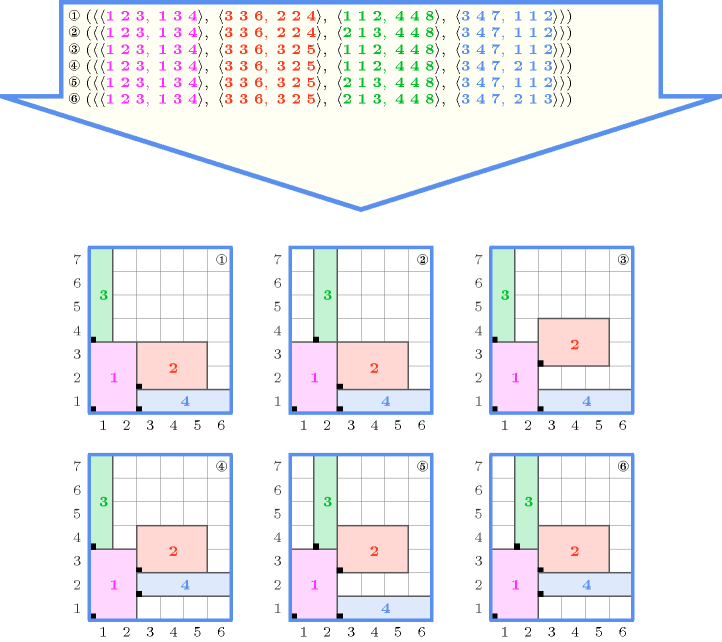Typical
 $|\mathrm{𝙾𝚁𝚃𝙷𝙾𝚃𝙾𝙿𝙴}|>1$ $\mathrm{𝙾𝚁𝚃𝙷𝙾𝚃𝙾𝙿𝙴}.\mathrm{𝚜𝚒𝚣}>0$ $|\mathrm{𝙾𝚁𝚃𝙷𝙾𝚃𝙾𝙿𝙴𝚂}|>1$
Symmetries
• Items of $\mathrm{𝙾𝚁𝚃𝙷𝙾𝚃𝙾𝙿𝙴𝚂}$ are permutable.

• Items of $\mathrm{𝙾𝚁𝚃𝙷𝙾𝚃𝙾𝙿𝙴𝚂}.\mathrm{𝚘𝚛𝚝𝚑}$ are permutable (same permutation used).

• $\mathrm{𝙾𝚁𝚃𝙷𝙾𝚃𝙾𝙿𝙴𝚂}.\mathrm{𝚘𝚛𝚝𝚑}.\mathrm{𝚜𝚒𝚣}$ can be decreased to any value $\ge 0$.

• One and the same constant can be added to the $\mathrm{𝚘𝚛𝚒}$ and $\mathrm{𝚎𝚗𝚍}$ attributes of all items of $\mathrm{𝙾𝚁𝚃𝙷𝙾𝚃𝙾𝙿𝙴𝚂}.\mathrm{𝚘𝚛𝚝𝚑}$.

Arg. properties

Contractible wrt. $\mathrm{𝙾𝚁𝚃𝙷𝙾𝚃𝙾𝙿𝙴𝚂}$.

Usage

The $\mathrm{𝚍𝚒𝚏𝚏𝚗}$ constraint occurs in placement and scheduling problems. It was for instance used for scheduling problems where one has to both assign each non-preemptive task to a resource and fix its origin so that two tasks, which are assigned to the same resource, do not overlap. When the resource is a set of persons to which non-preemptive tasks have to be assigned this corresponds to so called timetabling problems. A second practical application from the area of the design of memory-dominated embedded systems [Szymanek04] can be found in [SzymanekKuchcinski01]. Together with arithmetic and $\mathrm{𝚌𝚞𝚖𝚞𝚕𝚊𝚝𝚒𝚟𝚎}$ constraints, the $\mathrm{𝚍𝚒𝚏𝚏𝚗}$ constraint was used in [Szczygiel01] for packing more complex shapes such as angles. Figure 5.118.4 illustrates the angle packing problem on an instance involving 10 angles taken from [Szczygiel01].

##### Figure 5.118.4. A solution for the angle packing problem of items ${A}_{1}=\left[2,4,3,1\right]$, ${A}_{2}=\left[2,2,1,3\right]$, ${A}_{3}=\left[1,3,3,2\right]$, ${A}_{4}=\left[2,1,4,3\right]$, ${A}_{5}=\left[1,7,2,2\right]$, ${A}_{6}=\left[1,2,5,5\right]$, ${A}_{7}=\left[6,2,2,3\right]$, ${A}_{8}=\left[4,2,2,1\right]$, ${A}_{9}=\left[3,1,1,4\right]$, ${A}_{10}=\left[3,2,1,1\right]$.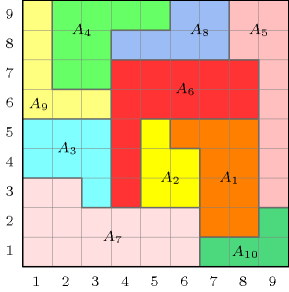One other packing problem attributed to S. Golomb is to find the smallest square that can contain the set of consecutive squares from $1×1$ up to $n×n$ so that these squares do not overlap each other (see the smallest rectangle area problem).

Remark

Unlike the definition of the Purpose slot the original paper [BeldiceanuContejean94] introducing the $\mathrm{𝚍𝚒𝚏𝚏𝚗}$ constraint imposes all orthotopes sizes to be different from 0. But it is convenient to allow variable sizes which can be assigned value 0 to model the fact that an orthotope can be skipped.

When we have segments (respectively rectangles) the $\mathrm{𝚍𝚒𝚏𝚏𝚗}$ constraint is referenced under the name $\mathrm{𝚍𝚒𝚜𝚓𝚘𝚒𝚗𝚝}\mathtt{1}$ (respectively $\mathrm{𝚍𝚒𝚜𝚓𝚘𝚒𝚗𝚝}\mathtt{2}$) in SICStus Prolog [Sicstus95]. When we have rectangles the $\mathrm{𝚍𝚒𝚏𝚏𝚗}$ constraint is also called $\mathrm{𝚍𝚒𝚏𝚏}\mathtt{2}$ in JaCoP. In MiniZinc (http://www.minizinc.org/) the $\mathrm{𝚍𝚒𝚏𝚏𝚗}$ constraint considers only rectangles.

It was shown in [Thiel04] that, finding out whether a non-overlapping constraint between a set of rectangles has a solution or not is NP-hard. This was achieved by reduction from sequencing with release times and deadlines.

In the two-dimensional case, when rectangles heights are all equal to one and when rectangles starts in the first dimension are all fixed, the $\mathrm{𝚍𝚒𝚏𝚏𝚗}$ constraint can be rewritten as a $𝚔_\mathrm{𝚊𝚕𝚕𝚍𝚒𝚏𝚏𝚎𝚛𝚎𝚗𝚝}$ constraint corresponding to a system of $\mathrm{𝚊𝚕𝚕𝚍𝚒𝚏𝚏𝚎𝚛𝚎𝚗𝚝}$ constraints derived from the maximum cliques of the corresponding interval graph.

Algorithm

Checking whether a $\mathrm{𝚍𝚒𝚏𝚏𝚗}$ constraint for which all variables are fixed is satisfied or not is related to the Klee's measure problem: given a collection of axis-aligned multi-dimensional boxes, how quickly can one compute the volume of their union. Then the $\mathrm{𝚍𝚒𝚏𝚏𝚗}$ constraint holds if the volume of the union is equal to the sum of the volumes of the different boxes.

A first possible method for filtering non zero size orthotopes is to use constructive disjunction. The idea is to try out each alternative of a disjunction (e.g., given two orthotopes ${o}_{1}$ and ${o}_{2}$ that should not overlap, we successively assume for each dimension that ${o}_{1}$ finishes before ${o}_{2}$, and that ${o}_{2}$ finishes before ${o}_{1}$) and to remove values that were pruned in all alternatives. For the two-dimensional case of $\mathrm{𝚍𝚒𝚏𝚏𝚗}$ a second possible solution used in [RochonDuVerdier92] is to represent explicitly the two-dimensional domain of the origin of each rectangle by a quadtree [Samet89] and to accumulate all forbidden regions within this data structure. As for conventional domain variables, a failure occurs when a two-dimensional domain get empty. A third possible filtering algorithm based on sweep is described in [BeldiceanuCarlsson01sweep].

The thesis of J. Nelissen [Nelissen94] considers the case where all rectangles have the same size and can be rotated from 90 degrees (i.e., the pallet loading problem.). For the $n$-dimensional case of $\mathrm{𝚍𝚒𝚏𝚏𝚗}$ a filtering algorithm handling the fact that two objects do not overlap is given in [BeldiceanuGuoThiel01].

##### Figure 5.118.5. A hard instance from [Nelissen94]: A solution for packing 99 rectangles of size $5×9$ into a rectangle of size $86×52$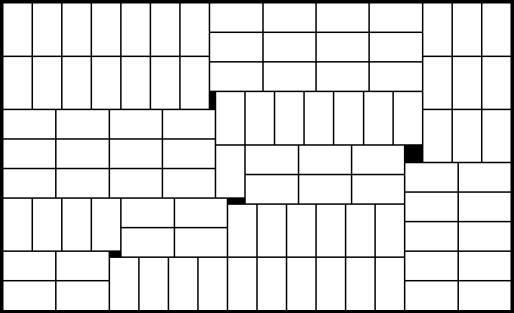Extensions of the non-overlapping constraint to polygons and to more complex shapes are respectively described in  [BeldiceanuGuoThiel01] and in [RibeiroCarravilla04]. Specialised propagation algorithms for the squared squares problem [BouwkampDuijvestijn92] (based on the fact that no waste is permitted) are given in [Gambini99a] and in [Gambini99b].

The $\mathrm{𝚌𝚞𝚖𝚞𝚕𝚊𝚝𝚒𝚟𝚎}$ constraint can be used as a necessary condition for the $\mathrm{𝚍𝚒𝚏𝚏𝚗}$ constraint. Figure 5.118.6 illustrates this point for the two-dimensional case. A first (respectively second) $\mathrm{𝚌𝚞𝚖𝚞𝚕𝚊𝚝𝚒𝚟𝚎}$ constraint is obtained by forgetting the $y$-coordinate (respectively the $x$-coordinate) of the origin of each rectangle occurring in a $\mathrm{𝚍𝚒𝚏𝚏𝚗}$ constraint. Parts (B) and (C) respectively depict the cumulated profiles associated with the projection of the rectangles depicted by part (A) on the $x$ and $y$ axes.

The $\mathrm{𝚌𝚞𝚖𝚞𝚕𝚊𝚝𝚒𝚟𝚎}$ constraint is a necessary but not sufficient condition for the two-dimensional case of the $\mathrm{𝚍𝚒𝚏𝚏𝚗}$ constraint. Figure 5.118.7 illustrates this point on an example taken from [Biro90] where one has to place the 8 rectangles ${R}_{1}$, ${R}_{2}$, ${R}_{3}$, ${R}_{4}$, ${R}_{5}$, ${R}_{6}$, ${R}_{7}$, ${R}_{8}$ of respective size $5×2$, $8×2$, $6×1$, $5×1$, $2×1$, $3×1$, $2×2$ and $1×2$ in a big rectangle of size $12×4$. As shown by Figure 5.118.7 there is a cumulative solution where ${R}_{8}$ is split in two parts but M. Hujter proves in [Hujter90] that there is no solution where no rectangle is split.

##### Figure 5.118.6. Looking from the perspective of the $\mathrm{𝚌𝚞𝚖𝚞𝚕𝚊𝚝𝚒𝚟𝚎}$ constraint in a two-dimensional rectangles placement problem: projecting the three rectangles of (A) on the $x$ axis (B) and on the $y$ axis (C)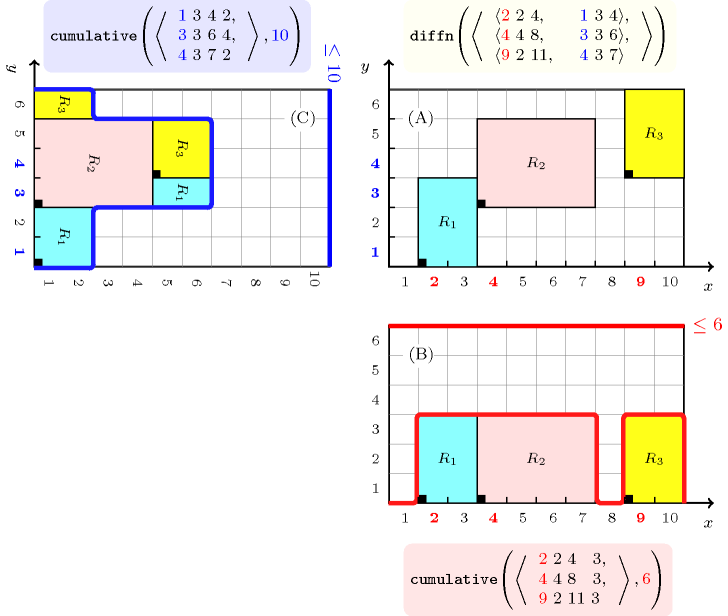##### Figure 5.118.7. Illustrating the necessary but not sufficient placement condition: the rectangle ${R}_{8}$ is split in two parts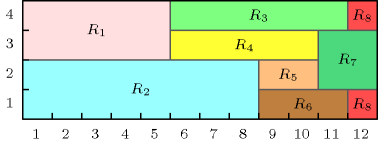In the context of $n$ parallelepipeds that have to be packed [GehringMenschnerMeyer90], [LiCheng90] within a box of sizes $X×Y×Z$ one can proceed as follows for stating three $\mathrm{𝚌𝚞𝚖𝚞𝚕𝚊𝚝𝚒𝚟𝚎}$ constraints. The ${i}^{th}$ (with $i\in \left[1,n\right]$) parallelepiped is described by the following attributes:

• ${\mathrm{𝑜𝑥}}_{i}$, ${\mathrm{𝑜𝑦}}_{i}$, ${\mathrm{𝑜𝑧}}_{i}$ (with $i\in \left[1,n\right]$) the coordinates of its origin on the $x$, $y$ and $z$-axes.

• ${\mathrm{𝑠𝑥}}_{i}$, ${\mathrm{𝑠𝑦}}_{i}$, ${\mathrm{𝑠𝑧}}_{i}$ (with $i\in \left[1,n\right]$) its sizes on the $x$, $y$ and $z$-axes.

• ${\mathrm{𝑝𝑥}}_{i}$, ${\mathrm{𝑝𝑦}}_{i}$, ${\mathrm{𝑝𝑧}}_{i}$ (with $i\in \left[1,n\right]$) the surfaces of its projections onto the planes $yz$, $xz$, and $xy$ respectively equal to ${\mathrm{𝑠𝑦}}_{i}{\mathrm{𝑠𝑧}}_{i}$, ${\mathrm{𝑠𝑥}}_{i}{\mathrm{𝑠𝑧}}_{i}$, and ${\mathrm{𝑠𝑥}}_{i}{\mathrm{𝑠𝑦}}_{i}$.

• ${𝑣}_{i}$ its volume (equal to ${\mathrm{𝑠𝑥}}_{i}{\mathrm{𝑠𝑦}}_{i}{\mathrm{𝑠𝑧}}_{i}$).

For the placement of $n$ parallelepipeds we get the following necessary conditions that respectively correspond to three $\mathrm{𝚌𝚞𝚖𝚞𝚕𝚊𝚝𝚒𝚟𝚎}$ constraints on the planes $yz$, $xz$, and $xy$:

$\left\{\begin{array}{c}\forall i\in \left[1,X\right]:{\sum }_{j\mid {\mathrm{𝑜𝑥}}_{j}\le i\le {\mathrm{𝑜𝑥}}_{j}+{\mathrm{𝑠𝑥}}_{j}-1}{\mathrm{𝑝𝑥}}_{j}\le YZ\\ \forall i\in \left[1,Y\right]:{\sum }_{j\mid {\mathrm{𝑜𝑦}}_{j}\le i\le {\mathrm{𝑜𝑦}}_{j}+{\mathrm{𝑠𝑦}}_{j}-1}{\mathrm{𝑝𝑦}}_{j}\le XZ\\ \forall i\in \left[1,Z\right]:{\sum }_{j\mid {\mathrm{𝑜𝑧}}_{j}\le i\le {\mathrm{𝑜𝑧}}_{j}+{\mathrm{𝑠𝑧}}_{j}-1}{\mathrm{𝑝𝑧}}_{j}\le XY\end{array}\right\$

Reformulation

Based on the fact that two orthotopes do not overlap if there exists at least one dimension where their projections do not overlap one can reformulate the $\mathrm{𝚍𝚒𝚏𝚏𝚗}$$\left(\mathrm{𝙾𝚁𝚃𝙷𝙾𝚃𝙾𝙿𝙴𝚂}\right)$ constraint as a disjunction of inequalities between the origin and the end attributes. In addition one has to link the origin, the size and the end attributes of each orthotope in each dimension.

If we consider the example described in the Example slot we get the following reformulation:

• $4=2+2$ (link between the origin, size and end in dimension 1 of the first orthotope),

• $4=1+3$ (link between the origin, size and end in dimension 2 of the first orthotope),

• $8=4+4$ (link between the origin, size and end in dimension 1 of the second orthotope),

• $6=3+3$ (link between the origin, size and end in dimension 2 of the second orthotope),

• $11=9+2$ (link between the origin, size and end in dimension 1 of the third orthotope),

• $7=4+3$ (link between the origin, size and end in dimension 2 of the third orthotope),

• $4\le 4\vee 8\le 2\vee 4\le 3\vee 6\le 1$ (non-overlapping between the first and second orthotopes),

• $4\le 9\vee 11\le 2\vee 4\le 4\vee 7\le 1$ (non-overlapping between the first and third orthotopes),

• $8\le 9\vee 11\le 4\vee 6\le 4\vee 7\le 3$ (non-overlapping between the second and third orthotopes).

Systems
Used in

implies: $\mathrm{𝚌𝚞𝚖𝚞𝚕𝚊𝚝𝚒𝚟𝚎}$ (implies one $\mathrm{𝚌𝚞𝚖𝚞𝚕𝚊𝚝𝚒𝚟𝚎}$ constraint for each dimension).

related: $\mathrm{𝚌𝚞𝚖𝚞𝚕𝚊𝚝𝚒𝚟𝚎}_\mathrm{𝚝𝚠𝚘}_𝚍$ ($\mathrm{𝚌𝚞𝚖𝚞𝚕𝚊𝚝𝚒𝚟𝚎}_\mathrm{𝚝𝚠𝚘}_𝚍$ is a necessary condition for $\mathrm{𝚍𝚒𝚏𝚏𝚗}$: forget one dimension when the number of dimensions is equal to 3), $\mathrm{𝚕𝚎𝚡}_\mathrm{𝚌𝚑𝚊𝚒𝚗}_\mathrm{𝚕𝚎𝚜𝚜}$, $\mathrm{𝚕𝚎𝚡}_\mathrm{𝚌𝚑𝚊𝚒𝚗}_\mathrm{𝚕𝚎𝚜𝚜𝚎𝚚}$ (lexicographic ordering on the origins of $\mathrm{𝚝𝚊𝚜𝚔𝚜}$, $\mathrm{𝚛𝚎𝚌𝚝𝚊𝚗𝚐𝚕𝚎𝚜}$, $...$), $\mathrm{𝚝𝚠𝚘}_\mathrm{𝚘𝚛𝚝𝚑}_\mathrm{𝚌𝚘𝚕𝚞𝚖𝚗}$, $\mathrm{𝚝𝚠𝚘}_\mathrm{𝚘𝚛𝚝𝚑}_\mathrm{𝚒𝚗𝚌𝚕𝚞𝚍𝚎}$.

specialisation: $\mathrm{𝚊𝚕𝚕}_\mathrm{𝚖𝚒𝚗}_\mathrm{𝚍𝚒𝚜𝚝}$ ($\mathrm{𝚘𝚛𝚝𝚑𝚘𝚝𝚘𝚙𝚎}$ replaced by $\mathrm{𝚕𝚒𝚗𝚎}\mathrm{𝚜𝚎𝚐𝚖𝚎𝚗𝚝}$, of same length), $\mathrm{𝚊𝚕𝚕𝚍𝚒𝚏𝚏𝚎𝚛𝚎𝚗𝚝}$ ($\mathrm{𝚘𝚛𝚝𝚑𝚘𝚝𝚘𝚙𝚎}$ replaced by $\mathrm{𝚟𝚊𝚛𝚒𝚊𝚋𝚕𝚎}$), $\mathrm{𝚌𝚞𝚖𝚞𝚕𝚊𝚝𝚒𝚟𝚎𝚜}$ ($\mathrm{𝚘𝚛𝚝𝚑𝚘𝚝𝚘𝚙𝚎}$ replaced by $\mathrm{𝚝𝚊𝚜𝚔}$ with $\mathrm{𝚖𝚊𝚌𝚑𝚒𝚗𝚎}$ $\mathrm{𝚊𝚜𝚜𝚒𝚐𝚗𝚖𝚎𝚗𝚝}$ and $\mathrm{𝚘𝚛𝚒𝚐𝚒𝚗}$ attributes), $\mathrm{𝚍𝚒𝚜𝚓𝚞𝚗𝚌𝚝𝚒𝚟𝚎}$ (orthotope replaced by $\mathrm{𝚝𝚊𝚜𝚔}$ of $\mathrm{𝚑𝚎𝚒𝚐𝚝𝚑}$ 1), $𝚔_\mathrm{𝚊𝚕𝚕𝚍𝚒𝚏𝚏𝚎𝚛𝚎𝚗𝚝}$ (when rectangles heights are all equal to 1 and rectangles starts in the first dimension are all fixed), $\mathrm{𝚕𝚎𝚡}_\mathrm{𝚊𝚕𝚕𝚍𝚒𝚏𝚏𝚎𝚛𝚎𝚗𝚝}$ ($\mathrm{𝚘𝚛𝚝𝚑𝚘𝚝𝚘𝚙𝚎}$ replaced by $\mathrm{𝚟𝚎𝚌𝚝𝚘𝚛}$).

Keywords
Arc input(s)

$\mathrm{𝙾𝚁𝚃𝙷𝙾𝚃𝙾𝙿𝙴𝚂}$

Arc generator
$\mathrm{𝑆𝐸𝐿𝐹}$$↦\mathrm{𝚌𝚘𝚕𝚕𝚎𝚌𝚝𝚒𝚘𝚗}\left(\mathrm{𝚘𝚛𝚝𝚑𝚘𝚝𝚘𝚙𝚎𝚜}\right)$

Arc arity
Arc constraint(s)
$\mathrm{𝚘𝚛𝚝𝚑}_\mathrm{𝚕𝚒𝚗𝚔}_\mathrm{𝚘𝚛𝚒}_\mathrm{𝚜𝚒𝚣}_\mathrm{𝚎𝚗𝚍}$$\left(\mathrm{𝚘𝚛𝚝𝚑𝚘𝚝𝚘𝚙𝚎𝚜}.\mathrm{𝚘𝚛𝚝𝚑}\right)$
Graph property(ies)
$\mathrm{𝐍𝐀𝐑𝐂}$$=|\mathrm{𝙾𝚁𝚃𝙷𝙾𝚃𝙾𝙿𝙴𝚂}|$

Arc input(s)

$\mathrm{𝙾𝚁𝚃𝙷𝙾𝚃𝙾𝙿𝙴𝚂}$

Arc generator
$\mathrm{𝐶𝐿𝐼𝑄𝑈𝐸}$$\left(\ne \right)↦\mathrm{𝚌𝚘𝚕𝚕𝚎𝚌𝚝𝚒𝚘𝚗}\left(\mathrm{𝚘𝚛𝚝𝚑𝚘𝚝𝚘𝚙𝚎𝚜}\mathtt{1},\mathrm{𝚘𝚛𝚝𝚑𝚘𝚝𝚘𝚙𝚎𝚜}\mathtt{2}\right)$

Arc arity
Arc constraint(s)
$\mathrm{𝚝𝚠𝚘}_\mathrm{𝚘𝚛𝚝𝚑}_\mathrm{𝚍𝚘}_\mathrm{𝚗𝚘𝚝}_\mathrm{𝚘𝚟𝚎𝚛𝚕𝚊𝚙}$$\left(\mathrm{𝚘𝚛𝚝𝚑𝚘𝚝𝚘𝚙𝚎𝚜}\mathtt{1}.\mathrm{𝚘𝚛𝚝𝚑},\mathrm{𝚘𝚛𝚝𝚑𝚘𝚝𝚘𝚙𝚎𝚜}\mathtt{2}.\mathrm{𝚘𝚛𝚝𝚑}\right)$
Graph property(ies)
$\mathrm{𝐍𝐀𝐑𝐂}$$=|\mathrm{𝙾𝚁𝚃𝙷𝙾𝚃𝙾𝙿𝙴𝚂}|*|\mathrm{𝙾𝚁𝚃𝙷𝙾𝚃𝙾𝙿𝙴𝚂}|-|\mathrm{𝙾𝚁𝚃𝙷𝙾𝚃𝙾𝙿𝙴𝚂}|$

Graph model

The $\mathrm{𝚍𝚒𝚏𝚏𝚗}$ constraint is expressed by using two graph constraints:

• The first graph constraint forces for each dimension and for each orthotope the link between the corresponding $\mathrm{𝚘𝚛𝚒}$, $\mathrm{𝚜𝚒𝚣}$ and $\mathrm{𝚎𝚗𝚍}$ attributes.

• The second graph constraint imposes each pair of distinct orthotopes to not overlap.

Parts (A) and (B) of Figure 5.118.8 respectively show the initial and final graph associated with the second graph constraint of the Example slot. Since we use the $\mathrm{𝐍𝐀𝐑𝐂}$ graph property, the arcs of the final graph are stressed in bold.

##### Figure 5.118.8. Initial and final graph of the $\mathrm{𝚍𝚒𝚏𝚏𝚗}$ constraint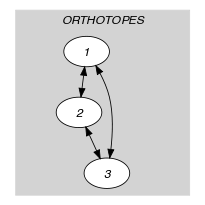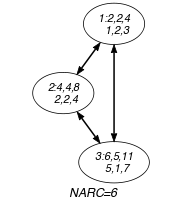(a) (b)
Signature

Since $|\mathrm{𝙾𝚁𝚃𝙷𝙾𝚃𝙾𝙿𝙴𝚂}|$ is the maximum number of vertices of the final graph of the first graph constraint we can rewrite $\mathrm{𝐍𝐀𝐑𝐂}$ $=$ $|\mathrm{𝙾𝚁𝚃𝙷𝙾𝚃𝙾𝙿𝙴𝚂}|$ to $\mathrm{𝐍𝐀𝐑𝐂}$ $\ge$ $|\mathrm{𝙾𝚁𝚃𝙷𝙾𝚃𝙾𝙿𝙴𝚂}|$. This leads to simplify $\underline{\overline{\mathrm{𝐍𝐀𝐑𝐂}}}$ to $\overline{\mathrm{𝐍𝐀𝐑𝐂}}$.

Since we use the $\mathrm{𝐶𝐿𝐼𝑄𝑈𝐸}\left(\ne \right)$ arc generator on the $\mathrm{𝙾𝚁𝚃𝙷𝙾𝚃𝙾𝙿𝙴𝚂}$ collection, $|\mathrm{𝙾𝚁𝚃𝙷𝙾𝚃𝙾𝙿𝙴𝚂}|·|\mathrm{𝙾𝚁𝚃𝙷𝙾𝚃𝙾𝙿𝙴𝚂}|-|\mathrm{𝙾𝚁𝚃𝙷𝙾𝚃𝙾𝙿𝙴𝚂}|$ is the maximum number of vertices of the final graph of the second graph constraint. Therefore we can rewrite $\mathrm{𝐍𝐀𝐑𝐂}$ $=$ $|\mathrm{𝙾𝚁𝚃𝙷𝙾𝚃𝙾𝙿𝙴𝚂}|·|\mathrm{𝙾𝚁𝚃𝙷𝙾𝚃𝙾𝙿𝙴𝚂}|-|\mathrm{𝙾𝚁𝚃𝙷𝙾𝚃𝙾𝙿𝙴𝚂}|$ to $\mathrm{𝐍𝐀𝐑𝐂}$ $\ge$ $|\mathrm{𝙾𝚁𝚃𝙷𝙾𝚃𝙾𝙿𝙴𝚂}|·|\mathrm{𝙾𝚁𝚃𝙷𝙾𝚃𝙾𝙿𝙴𝚂}|-|\mathrm{𝙾𝚁𝚃𝙷𝙾𝚃𝙾𝙿𝙴𝚂}|$. Again, this leads to simplify $\underline{\overline{\mathrm{𝐍𝐀𝐑𝐂}}}$ to $\overline{\mathrm{𝐍𝐀𝐑𝐂}}$.

Quiz

$\mathrm{𝚍𝚒𝚏𝚏𝚗}$: checking whether a ground instance holds or not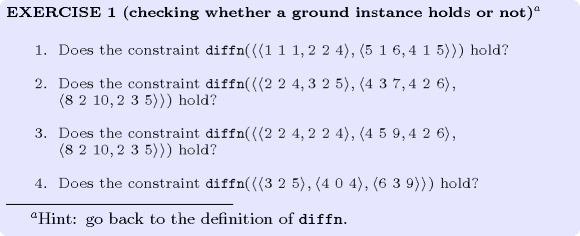$\mathrm{𝚍𝚒𝚏𝚏𝚗}$: finding all solutions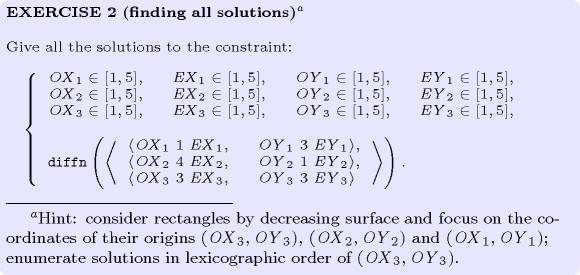$\mathrm{𝚍𝚒𝚏𝚏𝚗}$: finding the unique solution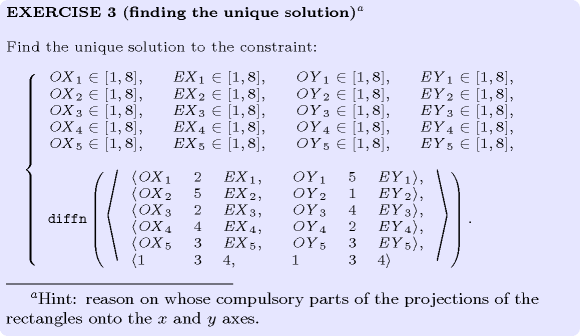$\mathrm{𝚍𝚒𝚏𝚏𝚗}$: degrees of violation for non-overlapping